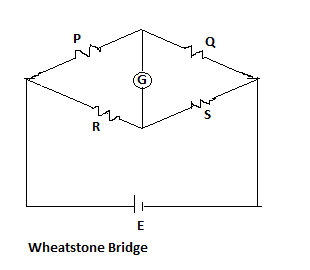# Measurement of Resistance - 1 - MCQs with answers

## Measurement of Resistance - 1 - MCQs with answers

Q1. Bridge circuits are used for the measurement of

A. Resistance
B. Inductance
C. Capacitance
D. All of these

Q2. Low resistance is the resistance of the order of

A. 1 ohm and less than 1 ohm
B. 1 ohm to 1 mega ohm
C. More than one ohm
D. None of these

ANSWER: A. 1 ohm and less than 1 ohm

Q3. The example of low resistance is/are

A. Resistance of armature windings of electrical machine
B. Resistance of series field winding of a dc machine
C. Resistances of shunts and lead wires
D. All of these

Q4. The accuracy in a bridge measurement depends on

A. Sensitivity of detector
B. Applied voltage
C. Accuracy of indicator
D. Both (a) and (b)

ANSWER: D. Both (a) and (b)

Q5. The high resistances are found in

A. Insulation resistance of cables and wires
B. Resistance of shunt field winding and the multipliers
C. Resistance of armature windings of electrical machine
D. Resistance of series field winding of a dc machine

ANSWER: A. Insulation resistance of cables and wires

Q6. A null type of bridge with dc excitation is commonly known as

A. Wheatstone bridge
B. Anderson bridge
C. Wien bridge
D. Schering bridge

Q7. In Wheatstone bridge method, the instrument used as null detector is

A. Ammeter
B. Voltmeter
C. Galvanometer
D. All of these

Q8. In a Wheatstone bridge method, the bridge is said to be balanced, when the current through the galvanometer is

A. 1 A
B. 0 A
C. Maximum
D. Half of the maximum value

Q9. The given figure shows the Wheatstone bridge method for measurement of unknown resistance (R).The balanced equation for Wheatstone bridge is given by

A. P/R = Q/S

B. P/S = Q/R

C. P/R = S/Q

D. R/P = Q/S

Q10. The sensitivity of Wheatstone bridge is defined as ratio of

A. Deflection of the galvanometer to the unit fractional change in the value of unknown resistance

B. Square of the deflection of the galvanometer to the unit fractional change in the value of unknown resistance

C. Deflection of the galvanometer to the twice of the unit fractional change in the value of unknown resistance

D. Unit fractional change in the value of unknown resistance to the deflection of the galvanometer